Definitions of Square Dance Calls and Concepts
Quadruple formation Concept [C3B]Index -->  Plus  |  A1  |  A2  |  C1  |  C2  |  C3A  |  C3B  |  C4  |  NOL  |
Definitions (Text Only) -->  Plus  |  A1  |  A2  |  C1  |  C2  |  C3A  |  C3B  |  C4  |  NOL  |
 Find call:

Quadruple formation Concept -- [C3B]
(author unknown) (upphovsman okänd) (autor neznámý)

 Language: \$B8@8l(B Språk: Jazyk: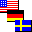All

Quadruple formations consist of four adjacent formation some of which may have positions occupied by phantoms. The given formation is usually a 4-dancer formation such as a Box, Column, Diamond, Line, or Wave. Work with the dancers and phantoms within your formation. Keep track of the phantoms and allow spaces for them upon completion of the call. When formation is a 4-dancer formation, there should be a total of 16 dancer | phantom positions before and after each call. EN: 10
Quadruple formations \$B\$O(B 4 \$B\$D\$NNY\$j9g\$C\$?(B formation \$B\$+\$i@.\$j(B, \$B\$=\$N\$&\$A\$N\$\$\$/\$D\$+\$K\$O(B phantom \$B\$N(B position \$B\$,\$"\$j\$^\$9(B. \$BM?\$(\$i\$l\$k(B formation \$B\$OIaDL(B, \$BNc\$(\$P(B Box, Column, Diamond, Line, \$B\$^\$?\$O(B Wave \$B\$N\$h\$&\$J(B 4 \$B?M\$N(B formation \$B\$G\$9(B. \$B<+J,\$N(B formation \$B\$G(B, \$B?M\$H(B phantom \$B\$H0l=o\$KF0\$-\$^\$9(B. Phantom \$B\$NF0\$-\$rDI\$C\$F(B, \$B%3!<%k\$N=*\$o\$j\$G\$O(B, phantom \$B\$N>l=j\$r6u\$1\$k\$h\$&\$K\$7\$^\$9(B. Formation \$B\$,(B 4 \$B?M\$N(B formation \$B\$N\$H\$-(B, \$BA4BN\$G\$O(B, \$B%3!<%k\$NA0\$H8e\$NN>J}\$H\$b(B, 16 \$B\$N?M\$^\$?\$O(B phantom \$B\$N(B position \$B\$,\$"\$j\$^\$9(B. JP: 10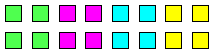Quadruple Box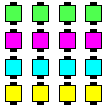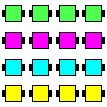Quadruple Line Quadruple Column

Notes: \$BCm Kommentarer: Poznámky: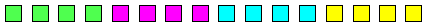(End-to-End) Quadruple Column|Line|Wave EN: 65 AddJapanese AddSwedish AddCzech (Infrequently used) EN: 67 (\$B\$^\$l\$K;H\$o\$l\$^\$9(B) JP: 67 AddSwedish AddCzech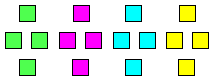Quadruple Diamond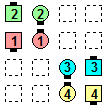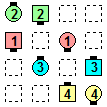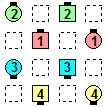before före před Quadruple Wave Swing Thru\$B\$NA0(B after efter po 1/2 by the Right\$B\$N8e(B after efter po 1/2 by the Left\$B\$N8e(B (done) (\$B=*\$o\$j(B) (klart) (hotovo)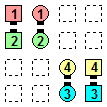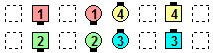before före před Quadruple Column Pass & Roll\$B\$NA0(B after efter po \$B8e(B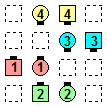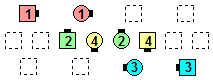before före před Quadruple Column Dixie Diamond\$B\$NA0(B after efter po \$B8e(B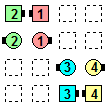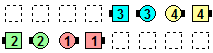before före před Quadruple Wave Turn The Key\$B\$NA0(B after efter po \$B8e(B

Note: Quadruple Wave Turn The Key is not the same as Split Phantom Waves Turn The Key. EN: 68
\$BCmJP: 68

In the former case, dancers work within their Wave of 4, and the Counter Rotate 1/4 is a Lockit;
whereas in the latter case, the Counter Rotate 1/4 is an 8-dancer (Parallel Lines) movement.
EN: 69

Sometimes, the outside formations can be at a 90° angle to the inside formations. For example, EN: 75
\$B30B&\$N(B formation \$B\$OFbB&\$N(B formation \$B\$KBP\$7\$F(B 90° \$B\$N3QEY\$H\$J\$k\$3\$H\$,\$"\$j\$^\$9(B. \$BNc\$(\$P(B, JP: 75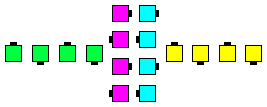Quadruple Wave

The formation may occasionally be a non-4-dancer formation. For example, EN: 70
Formation \$B\$O(B, \$B\$?\$^\$K\$O(B 4 \$B?M\$G\$J\$\$\$3\$H\$b\$"\$j\$^\$9(B. \$BNc\$(\$P(B, JP: 70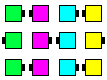Quadruple Wave of 3

Also possible, but rarely seen, are 6- or 8-dancer Quadruple formations (e.g., Quadruple Tall Six, Quadruple Hourglass, etc.). EN: 80
\$B\$^\$?(B, 6 \$B?M\$^\$?\$O(B 8 \$B?M\$N(B Quadruple formation (\$BNc\$(\$P(B, Quadruple Tall Six, Quadruple Hourglass, \$B\$J\$I(B) \$B\$,2DG=\$G\$9\$,(B, \$B\$[\$H\$s\$I;H\$o\$l\$^\$;\$s(B. JP: 80Choreography for Quadruple formation ConceptComments? Questions? Suggestions?